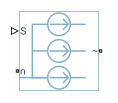# Controlled Current Source (Three-Phase)

Ideal three-phase controlled current source

• Library:
• Simscape / Electrical / Sources

•## Description

The Controlled Current Source (Three-Phase) block represents an ideal three-phase current source that is powerful enough to maintain the specified current through it regardless of the voltage across it. The output currents are [ia ib ic] = S, where S is a vector containing the numerical values presented at the physical signal port.

The figure shows the equivalent circuit for the expanded implementation of the Controlled Current Source (Three-Phase) block.To use the Controlled Current Source (Three-Phase) block as an abstracted current controller in an electrical drive, connect the conserving ports for the output current directly to the machine.

To choose the input type of this block, set the Input type parameter to `Instantaneous` or ```Sinusoidal magnitude and phase shift```. If you select `Instantaneous`, this block works only in time simulation mode. If you select `Sinusoidal magnitude and phase shift`, this block works in both time and frequency-time simulation modes. For more information, see Frequency and Time Simulation Mode.

To access the implementation with an expanded, three-phase port, double-click the block and set the Modeling option parameter to ```Expanded three-phase ports```.

## Ports

### Input

expand all

Physical signal input port associated with the control signal.

#### Dependencies

To enable this port, set Input type to `Instantaneous`.

Physical signal input port associated with the RMS current.

#### Dependencies

To enable this port, set Input type to ```Sinusoidal magnitude and phase shift```.

Physical signal input port associated with the phase shift, in radians.

#### Dependencies

To enable this port, set Input type to ```Sinusoidal magnitude and phase shift```.

### Conserving

expand all

Electrical conserving port associated with the neutral phase.

Expandable three-phase port.

#### Dependencies

To enable this port, set Modeling option to `Composite three-phase ports`.

Electrical conserving port associated with the a-phase output current.

#### Dependencies

To enable this port, set Modeling option to `Expanded three-phase ports`.

Electrical conserving port associated with the b-phase output current.

#### Dependencies

To enable this port, set Modeling option to `Expanded three-phase ports`.

Electrical conserving port associated with the c-phase output current.

#### Dependencies

To enable this port, set Modeling option to `Expanded three-phase ports`.

## Parameters

expand all

Whether to model composite or expanded three-phase ports.

Composite three-phase ports represent three individual electrical conserving ports with a single block port. You can use composite three-phase ports to build models that correspond to single-line diagrams of three-phase electrical systems.

Expanded three-phase ports represent the individual phases of a three-phase system using three separate electrical conserving ports.

Type of physical signal input. Choose between:

• `Instantaneous` — The output currents, [ia ib ic], are equal to the values of the input port S.

• ```Sinusoidal magnitude and phase shift``` — The output currents are equal to:

`$\begin{array}{l}{i}_{a}=\left(\sqrt{2}\text{\hspace{0.17em}}I\right)\mathrm{sin}\left(2\pi {F}_{rated}+ph\right)\\ {i}_{b}=\left(\sqrt{2}\text{\hspace{0.17em}}I\right)\mathrm{sin}\left(2\pi {F}_{rated}+ph-2\frac{\pi }{3}\right)\\ {i}_{c}=\left(\sqrt{2}\text{\hspace{0.17em}}I\right)\mathrm{sin}\left(2\pi {F}_{rated}+ph+2\frac{\pi }{3}\right)\end{array}$`

Rated electrical frequency. The value of this parameter must be equal to the value of the rated electrical frequencies of other sinusoidal sources in your model, if present.

#### Dependencies

To enable this parameter, set Input type to ```Sinusoidal magnitude and phase shift```.

## Version History

Introduced in R2018b